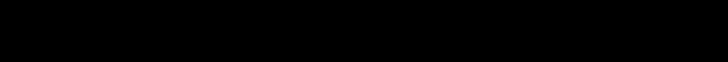Pattern Analysis Laboratory: RESEARCH
Department of Mathematics, Colorado State University
Mathematics and the Study of Patterns

The analysis of patterns in data has typically been a subject in statistics and engineering.  Recently, however, fundamental mathematical theory in areas such as differential geometry and topology has provided a new mathematical framework and insights for understanding large data sets residing in spaces of large ambient dimensions.  The research at the Pattern Analysis Laboratory emphasizes the transition of mathematical theory to efficient algorithms for exploring, understanding and modeling massive data sets.  It is remarkable how a mathematical framework can find applications across an array of seemingli  disparate problems.  Areas of interest include:

Low dimensional embeddings
Representation of data on Grassmann, Stiefel and flag manifolds
Geometric optimization
Computational geometry and topology

Geometric Motivation

Each member of a family of patterns shares features common to the other members in the family.  This correlation between images is a footprint of low dimensionality, or that a simpler representation exists.  The question now becomes how does the data sit geometrically in its ambient space and how can this coherence be exploited for data reduction.Applications
• pattern recognition, e.g., biological systems
• modeling of dynamical systems
• video and image processing
• signal processing
Topics of Interest
• Geometric data analysis including, Grassmannians, Flag manifolds
• Set to Set Pattern Classification
• Good projections,  Whitney's Theorem for data reduction, radial basis functions